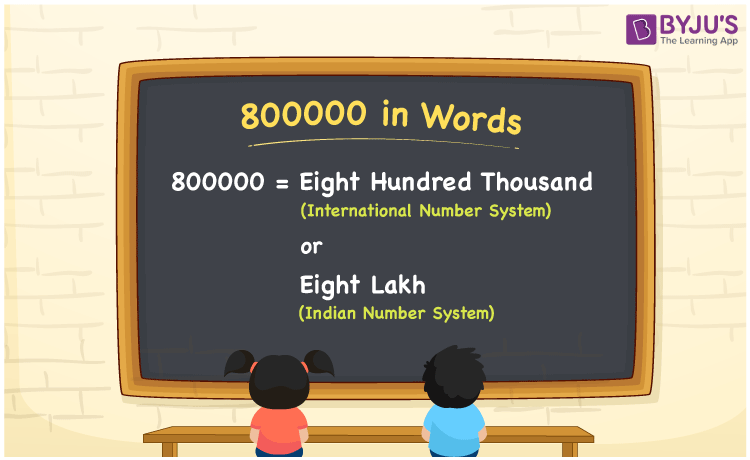# 800000 in Words

800000 in words is Eight Hundred Thousand (in International Number System) or Eight Lakh (in Indian Number System). If you bought a car worthRs. 800000, then you can write, you can write “I have bought a car worth Rupees Eight Lakh”. 800000 is a cardinal number as it represents a precise amount. Let us learn how to convert 800000 to words in this article.

 800000 in words International Number System Indian Number System Eight Hundred Thousand Eight Lakh Eight Hundred Thousand or Eight Lakh in numerical form 800000

## 800000 in English Words

To write numbers in words, we generally use the English alphabet and hence we can write 800000 in words as Eight Hundred Thousand or Eight Lakh.## How to Write 800000 in Words?

We can convert 800000 to words using a place value chart. The number 800000 has 6 digits. So, let us make a chart of place values up to 6 digits. The below table shows the place value chart for the number 800000.

 Lakhs Ten-Thousands Thousands Hundreds Tens Ones 8 0 0 0 0 0

Hence, we can write the expanded form as:

8 x Lakh + 0 x Ten-Thousand + 0 x Thousand + 0 x Hundred + 0 × Ten + 0 × One

= 8 x 100000 + 0 x 10000 + 0 x 1000 + 0 x 100 + 0 x 10 + 0 x 1

= 800000 + 0 + 0 + 0 + 0 + 0

= 800000

= Eight Hundred Thousand or Eight Lakh

Therefore, 800000 in words is written as Eight Hundred Thousand or Eight Lakh

Interesting way of writing 800000 in words

8 = Eight

80 = Eighty

800 = Eight Hundred

8000 = Eight Thousand

80000 = Eighty Thousand

800000 = Eight Hundred Thousand or Eight Lakh

Thus, the word form of the number 800000 is Eight Hundred Thousand or Eight Lakh.

800000 is a natural number that is succeeded by 799999 and preceded by 800001.

• 800000 in words – Eight Hundred Thousand or Eight Lakh
• Is 800000 an odd number? – No
• Is 800000 an even number? – Yes
• Is 800000 a perfect square number? – No
• Is 800000 a perfect cube number? – No
• Is 800000 a prime number? – No
• Is 800000 a composite number? – Yes

## Frequently Asked Questions on 800000 in Words

Q1

### How to express 800000 in words?

We can express 800000 in words as Eight Hundred Thousand or Eight Lakh.
Q2

### How do you write 800000 in words on a cheque?

On a cheque, we generally write 800000 in words as Eight Lakh rupees only.
Q3

### Write the spelling of 800000 in English.

The spelling of 800000 in English is Eight Hundred Thousand or Eight Lakh.